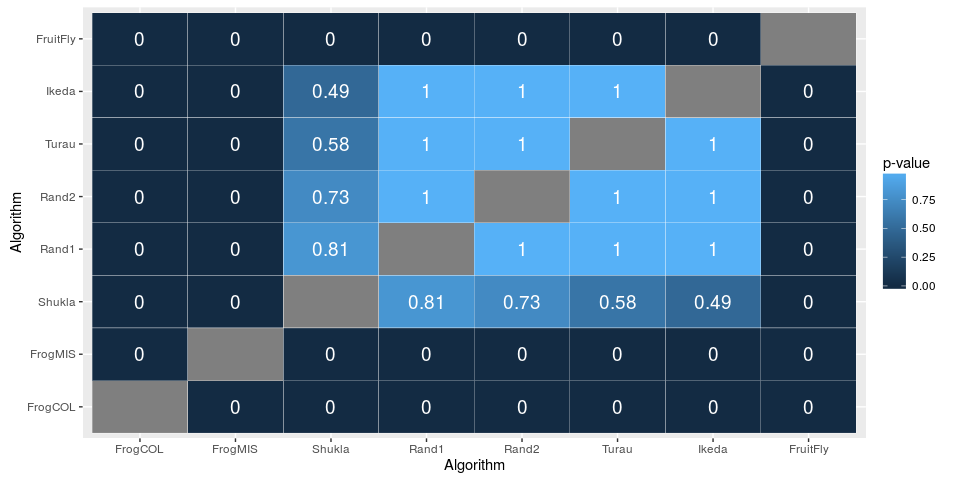# Statistical Assessment of the Differences

This vignette shows the use of the package scmamp to assess the statistical differences between the results obtained by a number of algorithms in different problems. This is a typical task in areas such as Machine Learning or Optimization, where algorithms are typically compared measuring their performance in different instances of problems, datasets, etc. However, a similar procedure may be used in other contexts.

The package and this vignette is based mainly on the papers García et al. (2010) and García and Herrera (2008), which is an extenstion of Demšar’s paper (Demšar (2006)).

If you are familiar with these papers and want a quick guide, jump to the last section of this document (Summary). Then, you can review the rest of the vignette for more details.

This vignette is divided into three different parts. The first reviews the global analysis for any algorithm behaving differently, and the other two are concerned with the post-hoc tests run in case not all the algorithms have the same performance. The second part shows how all pairwise tests can be conducted and the third how comparisons with respect to a control algorithm can be done. The election of the comparison will depend on the type of experimentation and the conclusions we want to draw.

As a guiding examples, we will use the results included in García and Herrera (2008) (Table 2) and the part of the results in Blum, Calvo, and Blesa (2015). These data is available in the package and can be loaded typing:

> library("scmamp")
> library("ggplot2")
> library("Rgraphviz")
> data(data_blum_2015)
> data(data_gh_2008)
> head(data.blum.2015)
##   Size Radius FruitFly Shukla Ikeda Turau Rand1 Rand2 FrogCOL FrogMIS
## 1 1000  0.049      223    213   214   214   214   212     246     226
## 2 1000  0.049      224    207   209   216   205   211     241     219
## 3 1000  0.049      219    206   215   214   209   213     243     221
## 4 1000  0.049      227    208   218   218   215   219     251     230
## 5 1000  0.049      231    218   210   212   211   217     243     239
## 6 1000  0.049      230    214   214   208   211   206     246     229
> head(data.gh.2008)
##             C4.5 k-NN(k=1) NaiveBayes Kernel   CN2
## Abalone*   0.219     0.202      0.249  0.165 0.261
## Adult*     0.803     0.750      0.813  0.692 0.798
## Australian 0.859     0.814      0.845  0.542 0.816
## Autos      0.809     0.774      0.673  0.275 0.785
## Balance    0.768     0.790      0.727  0.872 0.706
## Breast     0.759     0.654      0.734  0.703 0.714

These data represents the accuracy obtained by some algorithms in different datasets. Any other data can be used, provided that it is a named matrix or data.frame. For more details about how to load experimental results, please see the vignette concerning the loading and manipulation of data.

## Parametric vs. non-parametric

One of the very first things we need to decide is whether we can safely use parametric tests to assess the differences between algorithms. This is quite a tricky question, as using parametric tests when the assumptions hold yields a more powerful test, but the opposite may be true if they do not hold.

The classical parametric tests assume that the data is distributed according to a Gaussian distribution and, in most cases, that the variance is the same for all the samples. When this is true we can use these tests to have an increased power (compared with non parametric tests). Although there are statistical tests to check both the normality and the homocedasticity —many of them can be found in R, e.g., shapiro.test and bartlett.test—, they are not very powerful and this, together with the typically small samples, render them as non-effective tools.

For this reason, in this package we have included a couple of functions to visually valorate the assumptions of normality and homocedasticity. Note that, in some cases, there is no need to test this, as the data may be evidently non unimodal. An example of such situation is the data.blum.2015 data, where we have different types of problems, each with values in a different scale—You can check this by using the following functions to visualize the data.

The first plot we can crate is a density plot, using the function plotDensities. This function uses a kernel density estimation (KDE) of the distribution of the samples to visualize it.

> plotDensities (data=data.gh.2008, size=1.1)
## Warning: In density.default(data[, x], ...) :
##  extra argument 'size' will be disregarded

## Warning: In density.default(data[, x], ...) :
##  extra argument 'size' will be disregarded

## Warning: In density.default(data[, x], ...) :
##  extra argument 'size' will be disregarded

## Warning: In density.default(data[, x], ...) :
##  extra argument 'size' will be disregarded

## Warning: In density.default(data[, x], ...) :
##  extra argument 'size' will be disregarded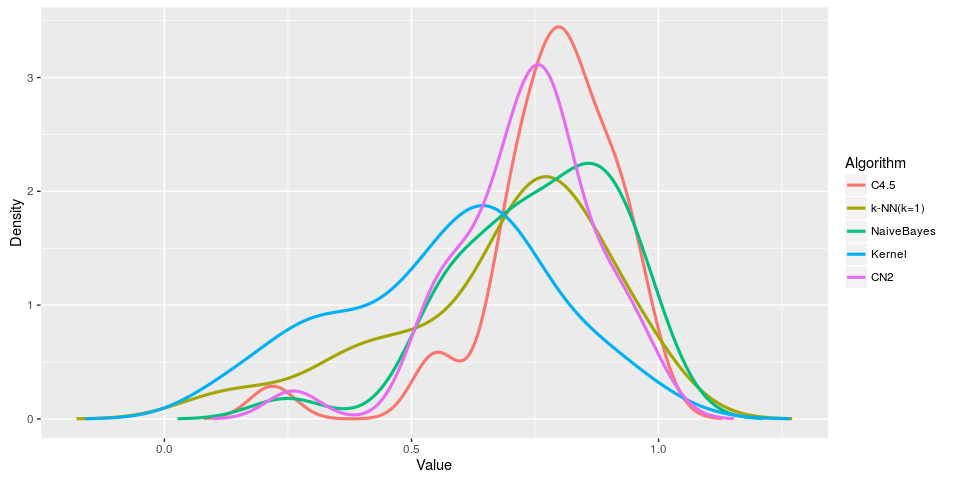The first and only mandatory argument is the matrix that includes the results for each algorithm. The plots are created using ggplot2, which is a powerfull tool to create plots in R. Morover, the result of this function is an object that can be further modified, as we will see in other plots. The function also accepts additional parameters that are directly passed to ggplot2’s function geom_line, which is the one that actually creates the lines; the size = 1.1 argument is an example of this.

In this plot we can see that most of the samples can be hardly regarded as normal, mainly due to their lack of symmetry and unimodality. Not only the assumption of normality does not hold, neither the assumption of equal variances seems to be true.

An additional kind of plot we can use to visually check the goodness of fit of the samples is the classical quantile-quantile plot, which represents the empirical and theoretical quantiles—assuming a Gaussian distribution. When all the points lay in the diagonal of the plot, both theoretical and empirical quantiles are equal and, thus, we can assume that the data can be approached with a Gaussian distribution. We can create these plots for each column using the qqplotGaussian function.

> qqplot <- qqplotGaussian (data.gh.2008[,"k-NN(k=1)"], size=5 , col="orchid")
> qqplot + theme_classic()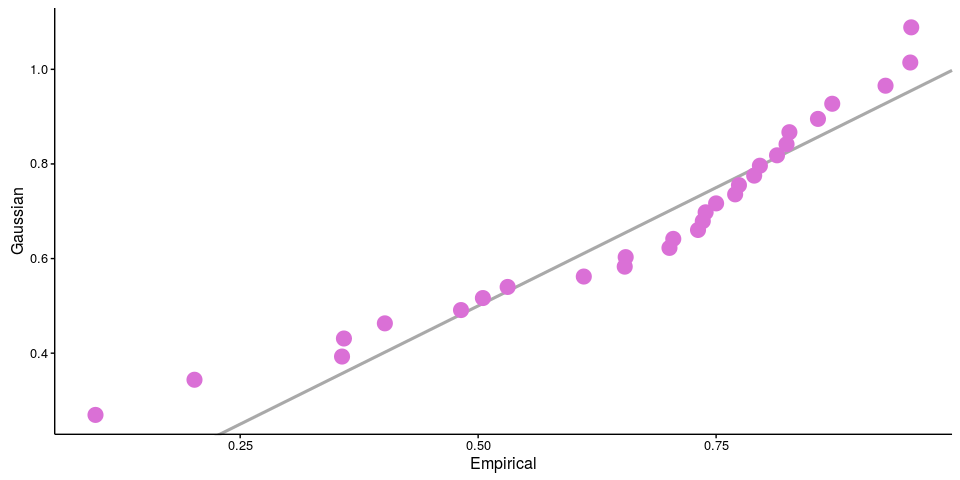As can be seen in the plot, there are regions where the sample points are away of the diagonal. This is particularly evident in the left part of the plot, due to the relatively long left tail of the empirical distribution. Additionally, the example shows one of the possible ways in which the result of the function can be modify to change its appearence. For the interested reader, there is an excelent book covering the use and the phylosophy behind ggplot2 (Wickham (2009)).

As a conclusion, in Demšar (2006) the author arguments against the use of parametric tests in the context of Machine Learning experiment analysis (see Demšar (2006), page 10); similar arguments can be applied to the evaluation of optimization algorithms.

## Testing for differences

Once the question parametric/non parametric is clear, the next step should be the use of a statistical test to check whether there are differences among the algorithms or not. In other words, determine if there is one or more algorithms whose performance can be regarded as significantly different.

In case the required assumptions are reasonably true, F-test for K population means (ANOVA) test can be used to assess the differences between the algorithms (the package include the function anovaTest to do this). However, as we have seen, in our running example it is clear that we cannot assume normality in the data. Therefore, in this example we will restrict the use of the package to non parametric methods.

This package includes two non parametric methods to compare multiple algorithms, the classical Friedman test (Friedman (1937)) and a modification by Iman and Davenport (Iman and Davenport (1980)). Although R’s base installation includes the former, we have reimplemented it in this as explained in Demšar (2006), page 11. This tests are available through functions friedmanTest and imanDavenportTest. In addition, the Friedman’s Aligned Rank Test and Quade Test presented in García et al. (2010) have been implemented—Note that this paper includes some errors in the computations due to some bugs in their code; for this reason, the results obtained with this package may not match those in the paper.

> friedmanTest(data.gh.2008)
##
##  Friedman's rank sum test
##
## data:  data.gh.2008
## Friedman's chi-squared = 39.647, df = 4, p-value = 5.121e-08
> imanDavenportTest(data.gh.2008)
##
##  Iman Davenport's correction of Friedman's rank sum test
##
## data:  data.gh.2008
## Corrected Friedman's chi-squared = 14.309, df1 = 4, df2 = 116,
## p-value = 1.593e-09
> friedmanAlignedRanksTest(data.gh.2008)
##
##  Friedman's Aligned Rank Test for Multiple Comparisons
##
## data:  data.gh.2008
## T = 41.691, df = 4, p-value = 1.933e-08
> quadeTest(data.gh.2008)
##
##  Quade for Multiple Comparisons
##
## data:  data.gh.2008
## T = 10.802, df = 4, p-value = 1.772e-07

The obtained p-values indicate that we can safely reject the null hypothesis that all the algorithms perform the same. Therefore, we can proceed with the post-hoc test.

We have two options, comparing all the algorithms among them or comparing all with a control. The latter is the typical situation where we are comparing a new proposal with the state of the art algorithms while the former fits better in a review of existing methods.

## Pairwise differences

Once we have verified that not all the performances of the algorithms are the same, the next step is analyzing which are different. For that, we have different possibilities.

### Nemenyi post hoc test

In Demšar (2006) the author proposes the use of the Nemenyi test that compares all the algorithms pairwise. It is the non parametric equivalent to the Tukey post hoc test for ANOVA (which is also available through the tukeyPost function), and is based on the absolute difference of the average rankings of the classifiers. For a significance level $$\alpha$$ the test determines the critical difference (CD); if the difference between the average ranking of two algorithms is grater than CD, then the null hypothesis that the algorithms have the same performance is rejected. The function nemenyiTest computes the critical difference and all the pairwise differences.

> test <- nemenyiTest (data.gh.2008, alpha=0.05)
> test
##
##  Nemenyi test
##
## data:  data.gh.2008
## Critical difference = 1.1277, k = 5, df = 145
> test$diff.matrix ## C4.5 k-NN(k=1) NaiveBayes Kernel CN2 ## [1,] 0.000000 -1.1500000 -0.1000000 -2.233333 -1.0166667 ## [2,] -1.150000 0.0000000 1.0500000 -1.083333 0.1333333 ## [3,] -0.100000 1.0500000 0.0000000 -2.133333 -0.9166667 ## [4,] -2.233333 -1.0833333 -2.1333333 0.000000 1.2166667 ## [5,] -1.016667 0.1333333 -0.9166667 1.216667 0.0000000 > abs(test$diff.matrix) > teststatistic ## C4.5 k-NN(k=1) NaiveBayes Kernel CN2 ## [1,] FALSE TRUE FALSE TRUE FALSE ## [2,] TRUE FALSE FALSE FALSE FALSE ## [3,] FALSE FALSE FALSE TRUE FALSE ## [4,] TRUE FALSE TRUE FALSE TRUE ## [5,] FALSE FALSE FALSE TRUE FALSE As the code above shows, with a significance of $$\alpha = 0.05$$ any two algorithms with a difference in the mean rank above 1.128 will be regarded as non equal. The test also returns a matrix with all the pair differences, so it can be used to see for which pairs the null hypothesis is rejected. As an example, the performance of C4.5 and 1NN are different, but we cannot state that C4.5 and Naive Bayes have a different behaviour. In Demšar (2006) the author proposes a plot to visually check the differences, the critical differece plot. This kind of plot can be created using the plotCD function, which has two parameters, the data. matrix and the significance level. In the plot, those algorithms that are not joined by a line can be regarded as different. > plotCD (data.gh.2008, alpha=0.05, cex=1.25)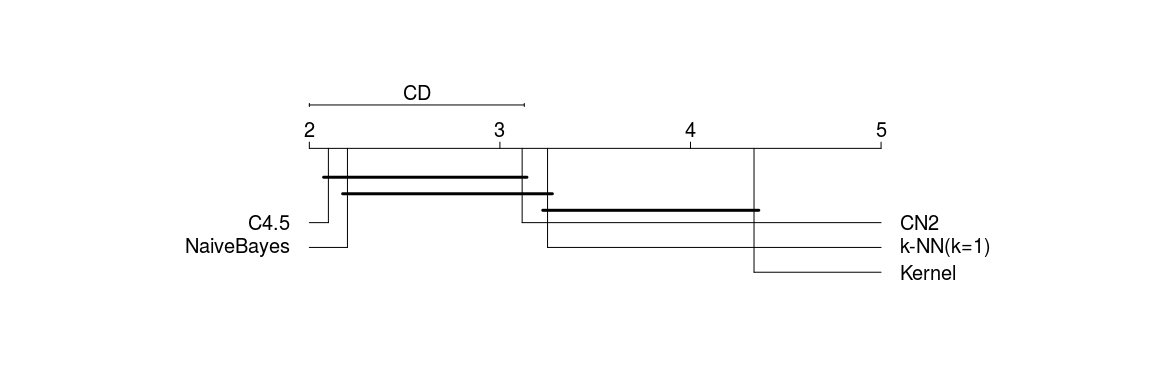> plotCD (data.gh.2008, alpha=0.01, cex=1.25)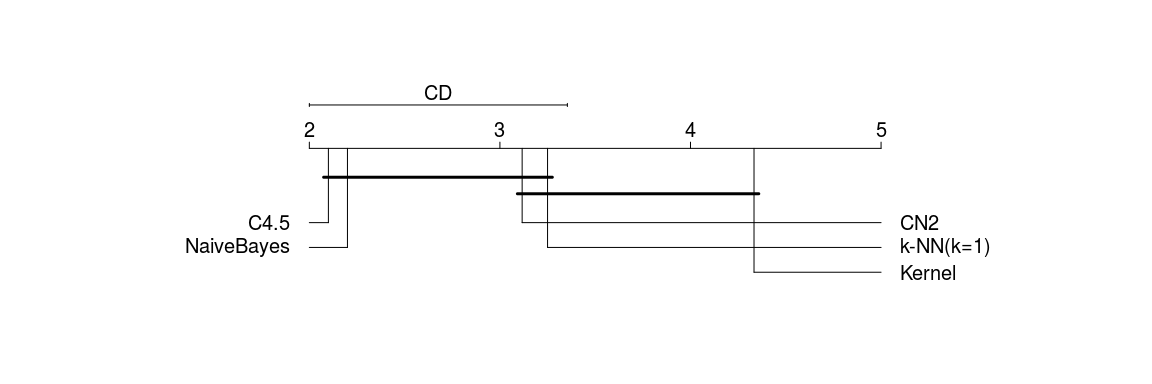Note that the text in the plot is defined in absolute size, while the rest is relative to the size of the plot. The default size (0.75) is tuned for a plot width of, roughly, 7 inches. In case the dimensions of the plot need to be bigger, the default size can be changed with the cex option, as in the example above (the dimension of these plots is 12x4 inches). This procedure is, among those implemented in the package, the one most conservative—i.e., the one with the less statistical power. Howerver, it provides an intiutive way to visualize the results. ### Corrected pairwise tests The second approach consists in using a classical test to assess all the pairwise differences between algorithms and then correct the p-values for multiple testing. In a parametric context the typicall election would be a paired t-test but, given that we cannot assume normality, we should use a non parametric test, such as Wilcoxon signed-rank test or the corresponding post hoc tests for Friedman, Friedman’s Aligned Ranks and Quade tests (see García and Herrera (2008), Section 2.1 and García et al. (2010), Section 5). The package includes the implementations of the post hoc tests mentioned in García et al. (2010) through functions friedmanPost, friedmanAlignedRanksPost and quadePost. > friedmanPost(data=data.gh.2008, control=NULL) ## C4.5 k-NN(k=1) NaiveBayes Kernel CN2 ## C4.5 NA 0.004848763 8.064959e-01 4.486991e-08 0.012763008 ## k-NN(k=1) 4.848763e-03 NA 1.011233e-02 7.963489e-03 0.743971478 ## NaiveBayes 8.064959e-01 0.010112334 NA 1.736118e-07 0.024744672 ## Kernel 4.486991e-08 0.007963489 1.736118e-07 NA 0.002880485 ## CN2 1.276301e-02 0.743971478 2.474467e-02 2.880485e-03 NA > quadePost(data=data.gh.2008, control=NULL) ## C4.5 k-NN(k=1) NaiveBayes Kernel CN2 ## C4.5 NA 0.08958006 0.9242154655 0.0004636398 0.18535894 ## k-NN(k=1) 0.0895800568 NA 0.1090468582 0.0713392870 0.70901220 ## NaiveBayes 0.9242154655 0.10904686 NA 0.0006596961 0.21895600 ## Kernel 0.0004636398 0.07133929 0.0006596961 NA 0.02951829 ## CN2 0.1853589437 0.70901220 0.2189560038 0.0295182898 NA > pv.matrix <- friedmanAlignedRanksPost(data=data.gh.2008, control=NULL) For the sake of flexibility, there is a special wrapper function, customPost, that allows applying any test. This function has a special argument, test, that has to be a function with, at least, two arguments, x and y, that performs the desired test. For more information, type ?customPost. The chosen test is applied to the $$\frac{k(k-1)}{2}$$ pairwise comparisons, where $$k$$ is the number of algorithms. Due to the multiple application of the test, some p-value correction method has to be used in order to control the familywise error rate. There are many general methods to correct this p-values, such as the well known Bonferroni procedure or Holm’s step-down method (Holm (1979)). However, these methods do not take into account the particular situation of pair-wise comparisons, where not any combination of null hypothesis can be true at the same time. As an example, suppose that we know that algorithms A and B are equal and, simultneously, A and C are also equal. Then, we cannot reject the hypothesis that A and C are equal. This problem was tackled by Juliet P. Shaffer (Shaffer (1986)). There are two procedures to correct the p-values, accoding to this paper. In the first one (sometimes called Shaffer static) the particular ordering of the null hypothesis is not taken into account and only the maximum number of simultaneous hypothesis is considered. The second one further limits the number of possible hypothesis by considering which particular hypothesis have been rejected. This increases the power of the method, but it is computationally very expensive. Instead of this procedure, in García and Herrera (2008), the authors propose to use Bergmann and Hommel’s method (Bergmann and Hommel (1988)). These procedures can be applied to a matrix of raw p-values using functions adjustShaffer and adjustBergmannHommel. > pv.matrix ## C4.5 k-NN(k=1) NaiveBayes Kernel CN2 ## C4.5 NA 0.001174850 3.341698e-01 2.843421e-10 1.845167e-02 ## k-NN(k=1) 1.174850e-03 NA 2.265711e-02 2.197407e-03 3.742777e-01 ## NaiveBayes 3.341698e-01 0.022657106 NA 9.226472e-08 1.643229e-01 ## Kernel 2.843421e-10 0.002197407 9.226472e-08 NA 7.793722e-05 ## CN2 1.845167e-02 0.374277740 1.643229e-01 7.793722e-05 NA > adjustShaffer(pv.matrix) ## C4.5 k-NN(k=1) NaiveBayes Kernel CN2 ## C4.5 NA 0.007049103 6.683396e-01 2.843421e-09 0.0738066768 ## k-NN(k=1) 7.049103e-03 NA 9.062842e-02 1.318444e-02 0.6683396134 ## NaiveBayes 6.683396e-01 0.090628422 NA 5.535883e-07 0.4929687992 ## Kernel 2.843421e-09 0.013184443 5.535883e-07 NA 0.0004676233 ## CN2 7.380668e-02 0.668339613 4.929688e-01 4.676233e-04 NA > pv.adj <- adjustBergmannHommel(pv.matrix) > pv.adj ## C4.5 k-NN(k=1) NaiveBayes Kernel CN2 ## C4.5 NA 0.007049103 6.683396e-01 2.843421e-09 0.0553550076 ## k-NN(k=1) 7.049103e-03 NA 6.797132e-02 8.789629e-03 0.6683396134 ## NaiveBayes 6.683396e-01 0.067971317 NA 5.535883e-07 0.1643229331 ## Kernel 2.843421e-09 0.008789629 5.535883e-07 NA 0.0003117489 ## CN2 5.535501e-02 0.668339613 1.643229e-01 3.117489e-04 NA The package also includes other correction methods, as we will see in the comparisons with a control algorithm. However, as these do not take into account the particular interactions between hypothesis, they are more restrictive approaches. Bergmann and Hommel’s correction is extremely expensive method—in computational terms. However, the structures required to perform the correction are stored in the disk and, thus, it is computationally tracktable up to 9 algorithms. ### Graphical representations Conversely to what happen with Nemenyi test, it makes no sense to draw a critical difference plot, since the critical differences are not constant throughout the comparisons. In absence of this intuitive plot, the package includes two types of plots to graphically display the results. The first function is drawAlgorithmGraph, which plots a graph where the algorithms are the nodes and two nodes are linked in the null hypothesis of being equal cannot be rejected. This function makes use of the Rgraphviz package, so it has to be installed in order to use this function. The package is currently in Bioconductor, so it can be installed as follows. > source("http://www.bioconductor.org/biocLite.R") > biocLite("Rgraphviz") The plot can incorporate information about each algorithm. In this case we will print the average ranking, in a similar way as in the critical difference plot. > r.means <- colMeans(rankMatrix(data.gh.2008)) > drawAlgorithmGraph(pvalue.matrix=pv.adj, mean.value=r.means, alpha=0.05, + font.size=10, node.width=3, node.height=1)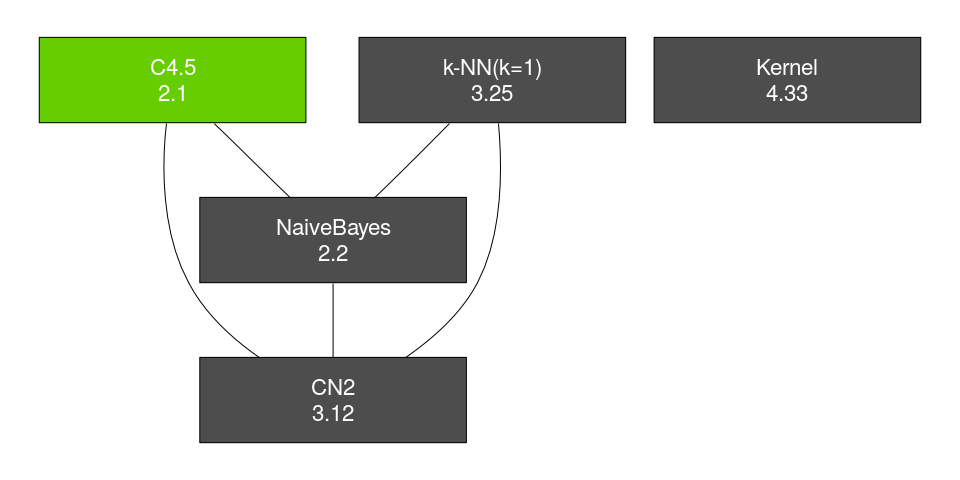In the code above we can see that there is a parameter called font.size, that can be used to change the font size to adapt it to the size of the plot (in a similar way as it happens in the critical difference plot). In addition to this, there is a number of parameters that can allow the user to customize the plot. The options are: • ... Special argument used to pass additional parameters. Its main use is changing the layout (see example bellow) • highlight It can be either 'min', 'max' or 'none', to highlight the node with the minimum value, the maximum value or none, respectively. • highlight.color A valid R color to fill the highlighted node • node.color A valid R color to fill the rest of the nodes • font.color A valid R color for the font • digits Number of digits to round the value included in the node • node.width Width of the node. By default it is set at 5 • node.height Height of the node. By default it is set at 2 The Rgraphviz package has a number of layouts that can be used to plot graphs (called 'dot', the one used by default, 'twopi', 'neato', 'circo' and 'fdp'). These layouts can be used including them right after the two first parameters. > r.means <- colMeans (rankMatrix(data.gh.2008)) > drawAlgorithmGraph (pvalue.matrix=pv.adj, mean.value=r.means, alpha=0.05, 'fdp', + highlight.color="red", node.color="white", font.color="black", + font.size=10, node.width=2, node.height=1)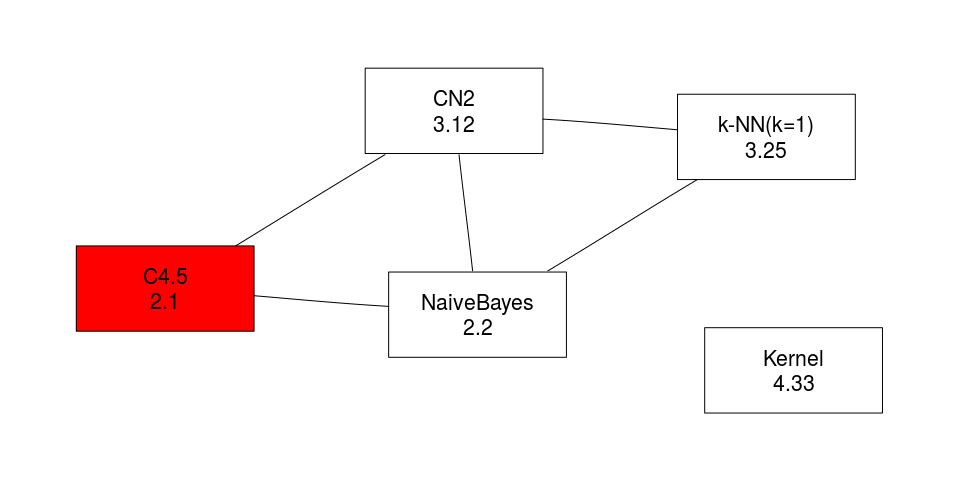This graph is the one corresponding to Bergmann and Hommel dynamic procedure. From its comparision with the previous one, we can check its increased power, as with the same $$\alpha$$ it rejects two more hypothesis, namely, that CN2 is equal to Naive Bayes and C4.5. The second plot can be used to directly visualize the p-value matrix generated when doing all the pairwise comparisons. The function that creates such a plot is plotPvalues. > plt <- plotPvalues(pvalue.matrix=pv.adj, + alg.order=order(r.means, decreasing=FALSE)) > plt + + labs(title="Corrected p-values using Bergmann and Hommel procedure") + + scale_fill_gradientn("Corrected p-values" , colours = c("skyblue4" , "orange")) ## Scale for 'fill' is already present. Adding another scale for 'fill', ## which will replace the existing scale.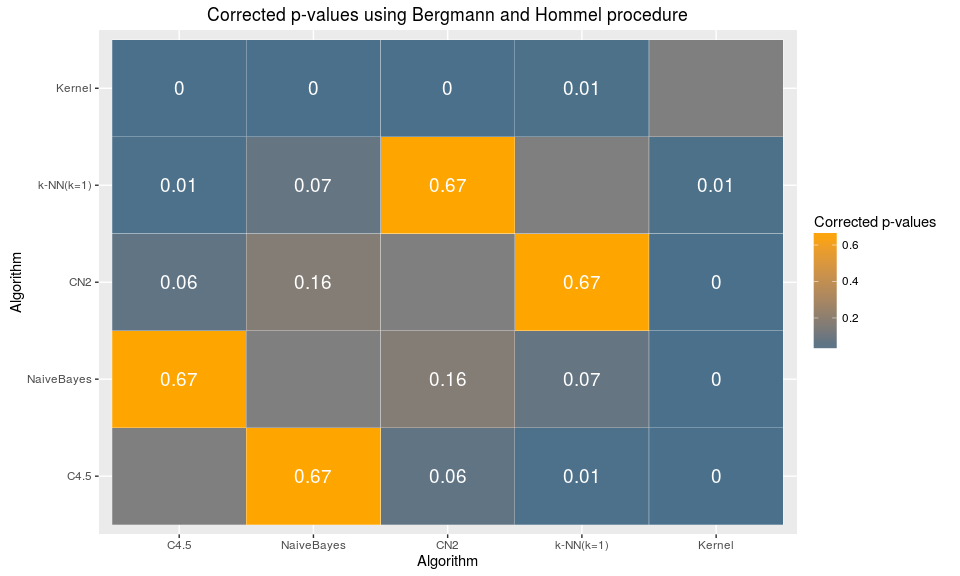The code above also shows how to modify some aesthetic aspects using ggplot2. ## Comaprison with a control In some experimentations we will be interested in comparing a set of algorithms with a control one—our proposal, typically. All the tests presented in the previous section can be also used in this case, fixing the control parameter to one of the algorithms in the data. When this parameter is not fixed —or set as NULL—, all the pairwise comparisons are performed, but when it takes a (valid) value, all the algorithms are compared with a reference. > friedmanAlignedRanksPost(data.gh.2008, control = "NaiveBayes") ## C4.5 k-NN(k=1) NaiveBayes Kernel CN2 ## [1,] 0.3341698 0.02265711 NA 9.226472e-08 0.1643229 > pv <- quadePost(data.gh.2008, control = 2) As can be seen in the code above, the reference can be set either using the column name or its index. The values computed in this way can be corrected to cope with the problem of multiple testing. However, in this case, using Shaffer and Brgmann and Hommel procedures makes no sense, as we do not have all the comparisons. Instead, we can use any of the methods listed in García et al. (2010). Some of these are implemented in the package and other are available through R’s p.adjust function. In particular, the methods implemented are: > adjustHolland(pvalues=pv) ## C4.5 k-NN(k=1) NaiveBayes Kernel CN2 ## [1,] 0.2562478 NA 0.2562478 0.2562478 0.7090122 > adjustFinner(pvalues=pv) ## C4.5 k-NN(k=1) NaiveBayes Kernel CN2 ## [1,] 0.2562478 NA 0.2562478 0.2562478 0.7090122 > adjustRom(pvalues=pv, alpha=0.05) ## C4.5 k-NN(k=1) NaiveBayes Kernel CN2 ## [1,] 0.2805656 NA 0.2805656 0.2805656 0.7090122 > adjustLi(pvalues=pv) ## Warning in adjustLi(pvalues = pv): The highest p-value is above 0.05. In ## such a situation the method is far too conservative, so consider using ## another method. ## C4.5 k-NN(k=1) NaiveBayes Kernel CN2 ## [1,] 0.2353852 NA 0.2725935 0.196892 0.7090122 ## Comparisons by groups of problems In some empirical evaluations we may be interested in analyzing the results obtained in different groups of instances. For example, in the case of the data from Blum, Calvo, and Blesa (2015) we may be interested in evaluating the algorithms in each problem size (100, 1000 and 5000). Computing the p-value in such an scenario is as simple as with a single group, but the correction of the p-values is by no means trivial, as all the comparisons should be considered. This is particularly complex in Shaffer and Bergmann and Hommel corrections, as the information about multiple pairwise comparisons has to be introduced. ## Global functions With the aim at further simplifying the use of the package we have defined two wrapper functions, multipleComparisonsTest, to perform the multiple comparison tests and postHocTest to run the individual comparisons. Both methods can be used either grouping the problems or using the whole dataset. Note that, in case all the problems are grouped the number of tests performed increases and, thus, the global number of tests should be considered when the p-values are adjusted. In the next section there are some example of the use of these functions, so here we will briefly descibe the main common arguments. Further information about the functions can be obtained from their help pages. • data A matrix or data.frame containing the algorithm results. The matrix can contain additional information (such as that used for grouping problems). • algorithms A vector (of, at least, size 2) with either the name or the indices of the columns in data that contain the observations that have to be compared. If this parameter is not passed (or if it is NULL), then all the columns except those used for grouping problems are regarded as algorithm columns. • group.by A vector with either the name or the indices of the columns in data that have to be used to group the problems. For each combination of values in these columns a test (or set of tests) is performed. • test Either a function or a string indicating the test to be used. The options and the type of function required depend on the function considered. For a complete list of options and the definition of the function please check the help page of each function. • correct Either a function or a string indicating the type of correction that has to be performed. The complete list of options and the type of functions required can be consulted in the help pages. Note that, for pairwise comparisons, Shaffer’s and Bergmann and Hommel’s corrections can be used, but only if the problems are not grouped. In case they are grouped, there are additional test repetitions that have to be accounted for and, thus, these methods have to be adapted. So far the package does not include this option and, thus, any of the other, general methods have to be used. • alpha Alpha value used only for Rom’s correction. • ... Additional parameters to be passed to either the test or the correct functions. In the case of postHocTest, these arguments are also passed to the rankMatirx function that computes the ranks of the data. Therefore, this can be used to change the default behaviour that ranks as 1 the highest value. To rank first the lowest value you can add the decreasing=FALSE option to the call. In the case of the postHocTest function, there are four additional parameters: • control This argument can be the index or the name of the column that has to be used as reference, min or max. In the last two options, for each comparison, the algorithm with the minimum/maximum summarized value is used as reference. If NULL (or not provided), all the pairwise comparisons are performed. • use.rank If TRUE, the rank of the algorithms is returned and used, in case control is set at min or max, to determine the control algorithm. If FALSE, the value in data is used instead. • sum.fun Function to be used to summarize the data. By default, the average value is used. This function also recieves the additional arguments passed to the function, so additional parameters can be passed to this function. Regarding the output of the functions, it depends on whether the problems are grouped or not. In the case of multipleComparisonsTest function, if the data is not grouped the result is an htest object, as any of the functions that performs this type of test. If the data is grouped, then the output is a matrix with the p-values (raw and adjusted) obtained for each group. In the case of postHocTest, in both cases the function outputs the summarized data (grouped or not), the raw p-values and the corrected p-values. In case the data is grouped and all the pairwise comparisons are performed, then the p-values are in a three dimensional array, being the last dimension the group to which the p-values correspond. ## Summary This section shows a couple of examples of typical comparisons done in the context of algorithm comparisons. In the first one all the data is included in a single comparison while in the second the data will be grouped according the the problem features. The typical sequence of analysis includes, first, testing the presence of any algorithm that behaves differently, using a test that compares simultaneously all the algorithms. Then, provided that the null hypothesis is rejected, a post hoc can be conducted. In case we can designate a control method, then the rest are tested against the control; in any other case, all the pairwise comparisons are performed. For the first example we will use the dataset from García and Herrera (2008). > alpha <- 0.05 > data <- data.gh.2008 > > friedmanTest(data) ## ## Friedman's rank sum test ## ## data: data ## Friedman's chi-squared = 39.647, df = 4, p-value = 5.121e-08 Alternatively, we can use any of the other methods implemented (e.g., imanDavenportTest or quadeTest), or the wrapper function multipleComparisonTest: > multipleComparisonTest(data=data, test="iman") ## ## Iman Davenport's correction of Friedman's rank sum test ## ## data: data.multipleComparisonTest ## Corrected Friedman's chi-squared = 14.309, df1 = 4, df2 = 116, ## p-value = 1.593e-09 Provided that the p-value obtained is below $$\alpha$$, if we have no control method then we can proceed with all the pairwise comparisons using the postHocTest wrapper function —alternatively you can use directly the functions that implement all the tests and corrections. In this case we can select any test for the comparisons. For the p-value correction, any method can be used, but in this particular case it is advisable using Bergman Hommel’s procedure if the number of algorithms to compare is 9 or less and Shaffer’s method in case they are 10 or more. The reason is that these methods include the particularities of the pairwise comparisons in order to perform a less conservative correction, leading to statistically more powerfull methods. > post.results <- postHocTest(data=data, test="aligned ranks", correct="bergmann", + use.rank=TRUE) > post.results ##summary
##      C4.5 k-NN(k=1) NaiveBayes   Kernel      CN2
## [1,]  2.1      3.25        2.2 4.333333 3.116667
##
## $raw.pval ## C4.5 k-NN(k=1) NaiveBayes Kernel CN2 ## C4.5 NA 0.001174850 3.341698e-01 2.843421e-10 1.845167e-02 ## k-NN(k=1) 1.174850e-03 NA 2.265711e-02 2.197407e-03 3.742777e-01 ## NaiveBayes 3.341698e-01 0.022657106 NA 9.226472e-08 1.643229e-01 ## Kernel 2.843421e-10 0.002197407 9.226472e-08 NA 7.793722e-05 ## CN2 1.845167e-02 0.374277740 1.643229e-01 7.793722e-05 NA ## ##$corrected.pval
##                    C4.5   k-NN(k=1)   NaiveBayes       Kernel          CN2
## C4.5                 NA 0.007049103 6.683396e-01 2.843421e-09 0.0553550076
## k-NN(k=1)  7.049103e-03          NA 6.797132e-02 8.789629e-03 0.6683396134
## NaiveBayes 6.683396e-01 0.067971317           NA 5.535883e-07 0.1643229331
## Kernel     2.843421e-09 0.008789629 5.535883e-07           NA 0.0003117489
## CN2        5.535501e-02 0.668339613 1.643229e-01 3.117489e-04           NA
> alg.order <- order(post.results$summary) > plt <- plotPvalues(post.results$corrected.pval, alg.order=alg.order)
> plt + labs(title=paste("Corrected p-values using Bergmann and Hommel procedure",sep=""))
## Warning: Removed 5 rows containing missing values (geom_text).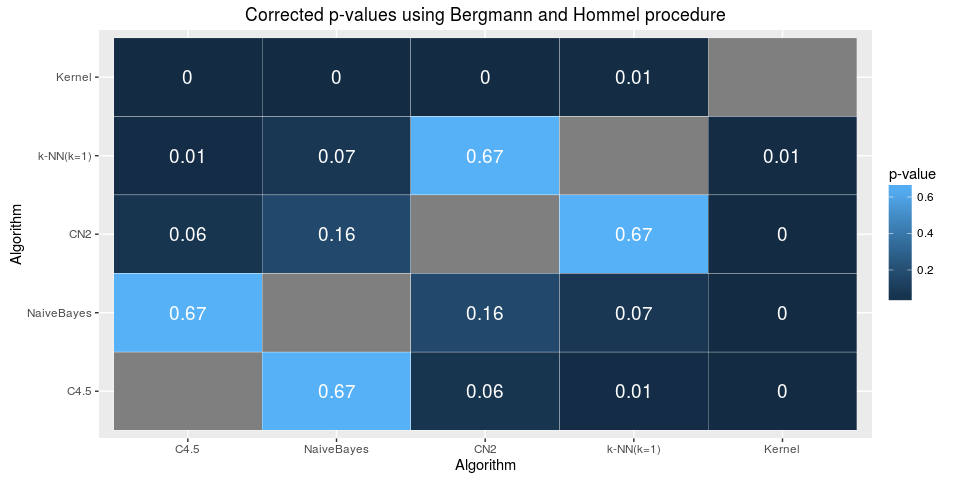> drawAlgorithmGraph(post.results$corrected.pval, mean.value=post.results$summary,
+                    alpha=alpha,  font.size=10)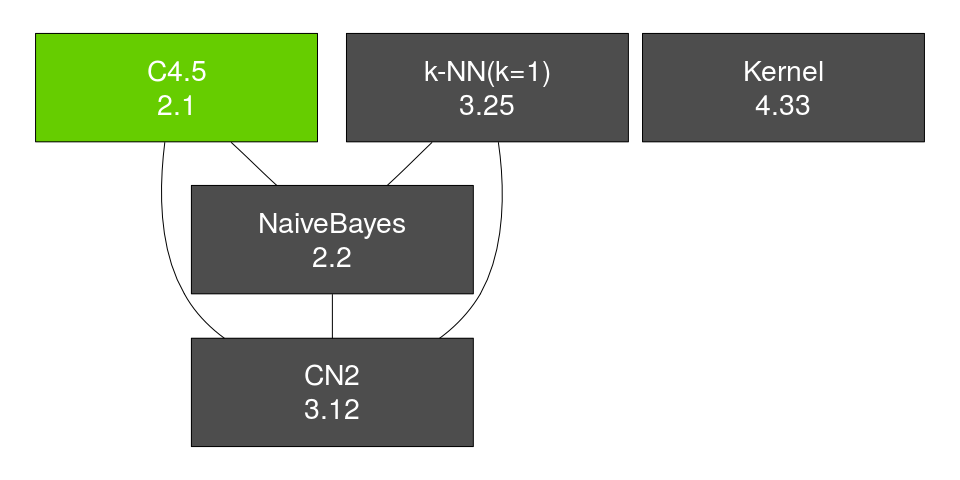For the second example we will use the dataset data.blum.2015, which contains two columns, Size and Radius, that allow us grouping the problems. First, we will search for differences in each comination of size and radius. Then, given that in all the cases the null hypothesis can be safely rejected, we will proceed with the comparison of all the methods with a control, the FrogCOL algorithm.

> data <- data.blum.2015
> group.by <- c("Size","Radius")
> multipleComparisonTest(data=data, group.by=group.by,
+                        test="quade", correct="finner")
##     Size Radius Raw_p-val_quade Corrected_p-val_finner
## 1   1000  0.049               0                      0
## 31  1000  0.058               0                      0
## 61  1000  0.067               0                      0
## 91  1000  0.076               0                      0
## 121 1000  0.085               0                      0
## 151 1000  0.094               0                      0
## 181 1000  0.103               0                      0
## 211 1000  0.112               0                      0
## 241 1000  0.121               0                      0
## 271 1000  0.134               0                      0
## 301  100  0.140               0                      0
## 331  100  0.143               0                      0
## 361  100  0.146               0                      0
## 391  100  0.149               0                      0
## 421  100  0.152               0                      0
## 451  100  0.155               0                      0
## 481  100  0.158               0                      0
## 511  100  0.161               0                      0
## 541  100  0.164               0                      0
## 571  100  0.169               0                      0
## 601 5000  0.024               0                      0
## 631 5000  0.036               0                      0
## 661 5000  0.048               0                      0
## 691 5000  0.060               0                      0
## 721 5000  0.072               0                      0
## 751 5000  0.084               0                      0
## 781 5000  0.096               0                      0
## 811 5000  0.108               0                      0
## 841 5000  0.120               0                      0
## 871 5000  0.134               0                      0
> control <- "FrogCOL"
> post.results <- postHocTest(data=data, group.by=group.by, control=control,
+                             test="aligned ranks", correct="rom", use.rank=FALSE)

The results can be used to create a LaTeX table where the results without significant differences with respect to our control are highlighted in italic and the best results in bold font.

> avg.val <- post.results$summary > best <- apply(avg.val, MARGIN=1, + FUN=function(x){ + m <- max(x[-(1:2)]) + return(c(FALSE, FALSE, x[-(1:2)]==m)) + }) > best <- t(best) > no.diff <- post.results$corrected.pval > alpha
## Warning in Ops.factor(left, right): '>' not meaningful for factors

## Warning in Ops.factor(left, right): '>' not meaningful for factors
> # The size and radius columns set as false
> no.diff[,1:2] <- FALSE
> no.diff[is.na(no.diff)] <- FALSE
> writeTabular(table=avg.val, format='f', bold=best, italic=no.diff,
+              hrule=c(0, 10, 20, 30), vrule=2, digits=c(0, 3, rep(2, 8)),
+              print.row.names = FALSE)
## \begin{tabular}{|ll|llllllll|}
## \hline
## Size & Radius & FruitFly & Shukla & Ikeda & Turau & Rand1 & Rand2 & FrogCOL & FrogMIS \\
## \hline
## 1000 & 0.049 & {\it 226.27} & 212.40 & 213.20 & 211.90 & 212.70 & 214.53 & {\bf 247.67} & {\it 227.60} \\
## 1000 & 0.058 & {\it 174.90} & 162.87 & 162.00 & 162.70 & 161.47 & 163.47 & {\bf 189.90} & {\it 176.93} \\
## 1000 & 0.067 & {\it 142.23} & 130.83 & 130.37 & 129.87 & 129.83 & 129.60 & {\bf 151.93} & {\it 140.57} \\
## 1000 & 0.076 & {\it 117.80} & 105.30 & 104.60 & 105.30 & 105.30 & 104.90 & {\bf 122.87} & {\it 114.13} \\
## 1000 & 0.085 & {\bf \it 99.43} & 87.43 & 85.87 & 86.70 & 86.73 & 87.07 & 102.37 & {\it 94.33} \\
## 1000 & 0.094 & {\bf \it 85.80} & 74.20 & 72.87 & 72.90 & 72.63 & 72.30 & 85.47 & {\it 79.33} \\
## 1000 & 0.103 & {\bf \it 75.57} & 63.17 & 62.77 & 62.13 & 63.23 & 62.30 & 74.20 & {\it 68.13} \\
## 1000 & 0.112 & {\bf \it 66.90} & 54.50 & 54.07 & 54.83 & 54.17 & 54.37 & 64.37 & {\it 58.20} \\
## 1000 & 0.121 & {\bf \it 59.53} & 47.97 & 47.43 & 47.17 & 47.03 & 47.60 & 56.50 & {\it 51.30} \\
## 1000 & 0.134 & 24.27 & 40.77 & 40.07 & 40.10 & 39.93 & 40.10 & {\bf 47.53} & {\it 43.10} \\
## \hline
## 100 & 0.140 & 27.80 & 25.63 & 25.70 & 25.90 & 26.17 & 26.10 & {\bf 31.07} & {\it 29.10} \\
## 100 & 0.143 & 26.80 & 25.43 & 25.60 & 25.53 & 25.17 & 25.73 & {\bf 30.53} & {\it 28.57} \\
## 100 & 0.146 & 26.57 & 24.90 & 25.37 & 25.80 & 25.07 & 25.30 & {\bf 29.93} & {\it 28.03} \\
## 100 & 0.149 & 25.80 & 24.47 & 23.97 & 24.40 & 23.87 & 24.40 & {\bf 29.10} & {\it 27.33} \\
## 100 & 0.152 & 25.03 & 23.77 & 23.57 & 23.57 & 23.83 & 23.87 & {\bf 28.47} & {\it 25.97} \\
## 100 & 0.155 & 24.13 & 23.27 & 22.87 & 23.00 & 22.63 & 22.90 & {\bf 27.30} & {\it 25.47} \\
## 100 & 0.158 & 24.03 & 22.47 & 22.63 & 22.50 & 22.40 & 22.90 & {\bf 27.33} & 24.57 \\
## 100 & 0.161 & 22.80 & 21.70 & 21.03 & 21.93 & 21.57 & 21.40 & {\bf 25.83} & 23.40 \\
## 100 & 0.164 & 22.40 & 20.80 & 20.67 & 20.90 & 20.93 & 21.43 & {\bf 25.57} & 22.93 \\
## 100 & 0.169 & 21.73 & 20.57 & 20.10 & 20.03 & 20.13 & 20.17 & {\bf 24.50} & 22.20 \\
## \hline
## 5000 & 0.024 & {\it 964.53} & 898.23 & 899.60 & 902.50 & 897.83 & 905.30 & {\bf 975.90} & {\it 972.40} \\
## 5000 & 0.036 & {\bf \it 515.83} & 459.30 & 453.87 & 452.97 & 455.57 & 456.60 & 502.00 & {\it 495.30} \\
## 5000 & 0.048 & {\bf \it 332.13} & 276.40 & 273.67 & 273.00 & 275.23 & 273.83 & 309.17 & {\it 294.10} \\
## 5000 & 0.060 & {\bf 42.03} & 185.60 & 182.30 & 183.43 & 184.73 & 183.00 & 210.90 & {\it 196.93} \\
## 5000 & 0.072 & {\bf 2.80} & 134.97 & 131.20 & 131.47 & 132.50 & 131.90 & 153.90 & {\it 139.87} \\
## 5000 & 0.084 & 0.27 & 102.03 & {\bf 99.87} & 99.60 & 99.37 & 99.83 & 117.90 & {\it 105.73} \\
## 5000 & 0.096 & 0.00 & 79.97 & 78.77 & 78.60 & 78.73 & 78.20 & {\bf 93.30} & {\it 82.57} \\
## 5000 & 0.108 & 0.00 & 64.50 & 63.73 & 63.40 & 63.33 & 63.10 & {\bf 75.83} & {\it 67.43} \\
## 5000 & 0.120 & 0.00 & 54.07 & 52.10 & 51.90 & 52.63 & 52.60 & {\bf 63.27} & 54.57 \\
## 5000 & 0.134 & 0.00 & 44.27 & 43.43 & 43.47 & 43.10 & 43.07 & {\bf 52.20} & {\it 45.47} \\
## \hline
## \end{tabular}

As an alternative analysis, we will compare, for each graph size, all the algorithms. Note that, in this case, as the data contains the Radius column that should not be included in the comparison, we have to specify the columns that contain the algorithms—or, alterantively, remove the column from the data.

> control <- NULL
> group.by <- "Size"
> post.results <- postHocTest(data=data, algorithms=3:10, group.by=group.by,
+                             control=control, test="aligned ranks", correct="holland",
+                             use.rank=TRUE)
>
> # Plot the matrix for the first group
> i <- 1
> alg.order <- order(post.results$summary[i,-1]) > plotPvalues(post.results$corrected.pval[, , i], alg.order=alg.order) 
## Warning: Removed 8 rows containing missing values (geom_text).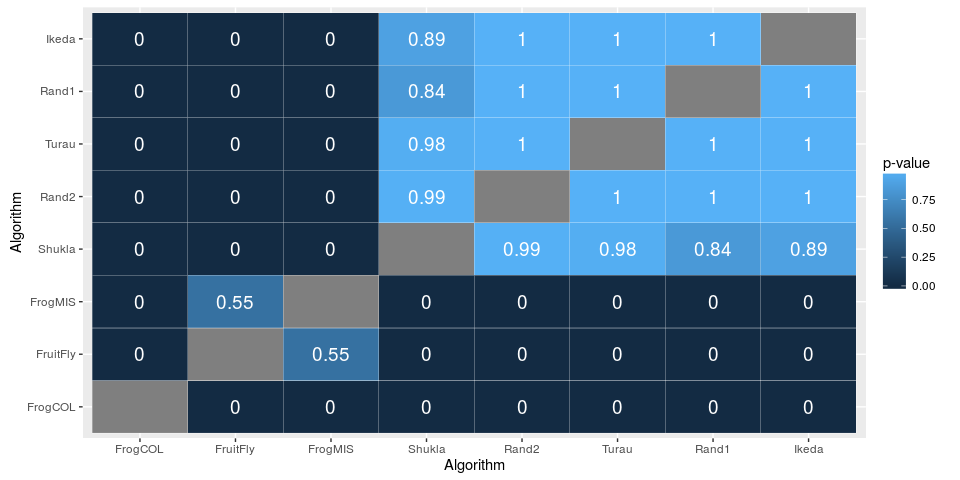> # Plot the matrix for the second group
> i <- 2
> alg.order <- order(post.results$summary[i,-1]) > plotPvalues(post.results$corrected.pval[, , i], alg.order=alg.order) 
## Warning: Removed 8 rows containing missing values (geom_text).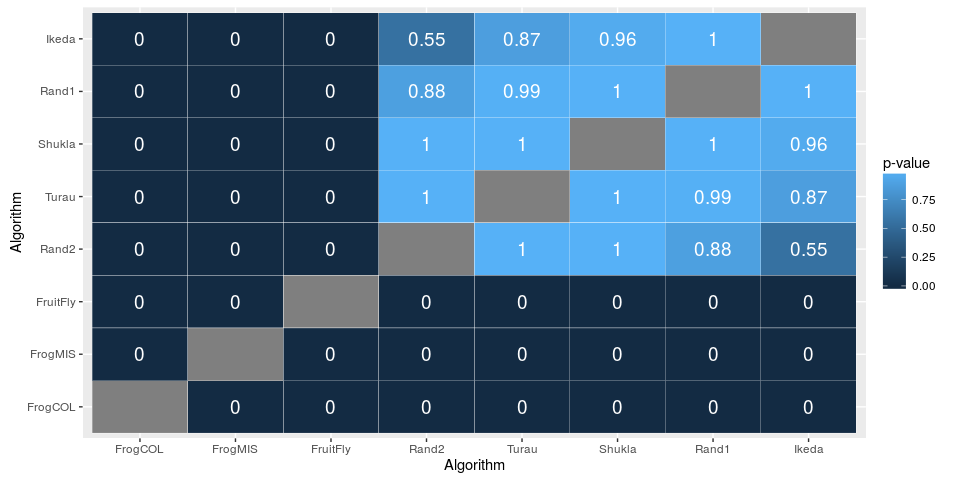> # Plot the matrix for the third group
> i <- 3
> alg.order <- order(post.results$summary[i,-1]) > plotPvalues(post.results$corrected.pval[, , i], alg.order=alg.order) 
## Warning: Removed 8 rows containing missing values (geom_text).